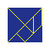# Should projection always change coordinate values?

366
3
06-26-2019 12:17 PMNew Contributor III

In the following code sample, why does point2/s2 change its values after being projected, but point4/s4 does not?

How do I get point4's coordinates to change?

The transformation property is null for both projTrans1 and projTrans3.

Thanks!

``````QueuedTask.Run(() =>
{
string wkt1 = "GEOGCS[\"GCS_WGS_1984\", DATUM[\"D_WGS_1984\", SPHEROID[\"WGS_1984\", 6378137.0, 298.257223563]], PRIMEM[\"Greenwich\", 0.0], UNIT[\"Grad\", 0.01570796326794897]]";

string wkt2 = "GEOGCS[\"GCS_WGS_1984\", DATUM[\"D_WGS_1984\", SPHEROID[\"WGS_1984\", 6378137.0, 298.257223563]], PRIMEM[\"Greenwich\", 0.0], UNIT[\"Degree\", 0.0174532925199433]]";

string wkt3 = "GEOGCS[\"GCS_WGS_1984\", DATUM[\"D_WGS_1984\", SPHEROID[\"WGS_1984\", 6378137.0, 298.257223563]], PRIMEM[\"Greenwich\", 0.0], UNIT[\"Degree\", 0.0174532925199433]]," +
"VERTCS[\"WGS_1984\", DATUM[\"D_WGS_1984\", SPHEROID[\"WGS_1984\", 6378137.0, 298.257223563]], PARAMETER[\"Vertical_Shift\", 0.0], PARAMETER[\"Direction\", 1.0], UNIT[\"Yard\", 0.9144]]";

string wkt4 = "GEOGCS[\"GCS_WGS_1984\", DATUM[\"D_WGS_1984\", SPHEROID[\"WGS_1984\", 6378137.0, 298.257223563]], PRIMEM[\"Greenwich\", 0.0], UNIT[\"Degree\", 0.0174532925199433]]," +
"VERTCS[\"WGS_1984\", DATUM[\"D_WGS_1984\", SPHEROID[\"WGS_1984\", 6378137.0, 298.257223563]], PARAMETER[\"Vertical_Shift\", 0.0], PARAMETER[\"Direction\", 1.0], UNIT[\"Meter\", 1.0]]";

SpatialReference pSR1 = SpatialReferenceBuilder.CreateSpatialReference(wkt1);
SpatialReference pSR2 = SpatialReferenceBuilder.CreateSpatialReference(wkt2);
SpatialReference pSR3 = SpatialReferenceBuilder.CreateSpatialReference(wkt3);
SpatialReference pSR4 = SpatialReferenceBuilder.CreateSpatialReference(wkt4);

MapPoint point1 = MapPointBuilder.CreateMapPoint(10, 20, 300, pSR1);
MapPoint point3 = MapPointBuilder.CreateMapPoint(10, 20, 300, pSR3);

ProjectionTransformation projTrans1 = ProjectionTransformation.Create(pSR1, pSR2, point1.Extent);
ProjectionTransformation projTrans3 = ProjectionTransformation.CreateWithVertical(pSR3, pSR4, point3.Extent);

MapPoint point2 = (MapPoint)GeometryEngine.Instance.ProjectEx(point1, projTrans1);
MapPoint point4 = (MapPoint)GeometryEngine.Instance.ProjectEx(point3, projTrans3);
string s2 = \$"X: {point2.X} Y: {point2.Y}  Z: {point2.Z}  M: {point2.M}";
string s4 = \$"X: {point4.X} Y: {point4.Y}  Z: {point4.Z}  M: {point4.M}";

// s2 = "X: 9 Y: 18  Z: 300  M: NaN"
// s4 = "X: 10 Y: 20  Z: 300  M: NaN"
});‍‍‍‍‍‍‍‍‍‍‍‍‍‍‍‍‍‍‍‍‍‍‍‍‍‍‍‍‍‍‍``````
3 RepliesbyEsri Notable Contributor

The first transformation converted from grads to degrees, even though the geographic/datum transformation wasn't set.

On the next reprojection, did you mean to use point1 or point3? Point1 doesn't have an input VCS, so there's no way to change the units.

MelitaNew Contributor III

Thanks Melita!

Thank you for catching the typo. Yes, line 25 should be projecting point3 not point1.
I've corrected my code above and reran my sample, but I still get the same result //s4 = "X: 10 Y: 20  Z: 300  M: NaN"

Should point4 have also converted from vertical yards to meters?

- JustinbyEsri Notable Contributor

I would hope so, but we have been going back-and-forth internally about when unit conversions should be done outside of actual transformations. I think we turned them off for vertical data because it was causing another problem elsewhere.